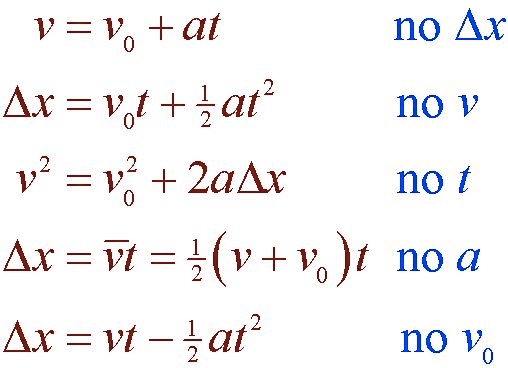# Special Case: Constant Acceleration

• For cases of constant acceleration, we can derive a set of "kinematic equations" that will allow us to solve for unknown quantities# Deriving the Kinematic Equations# Free Fall

• When the only force acting on an object is the force of gravity, we refer to object's motion as free fall

• This includes objects that have a non-zero initial velocity

# Air Resistance

• If we drop a ball and a sheet of paper, it is obvious they don't fall at the same rate

• If we could remove all the air from the room, however, we would find that they fall at the same rate

• We will analyze the motion of objects by neglecting air resistance (a form of friction) for the time being

# Acceleration Due to Gravity

• Near the surface of Earth, objects accelerate downward at a rate of 9.8m/s2

• We call this acceleration the acceleration due to gravity (g)

• More accurately, g is referred to as the gravitational field strength

• As you move further away from Earth, g decreases